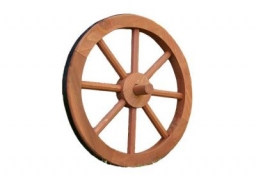# Circumference 22243

The circumference of the first wheel is 0.5 m larger than the circumference of the second wheel. The first wheel turns on the 36 m track as many times as the second on the 30 m track. Determine the rims of both wheels.

a =  3 m
b =  2.5 m

### Step-by-step explanation:Did you find an error or inaccuracy? Feel free to write us. Thank you!

Tips for related online calculators
Do you have a linear equation or system of equations and looking for its solution? Or do you have a quadratic equation?

#### Grade of the word problem:

We encourage you to watch this tutorial video on this math problem: